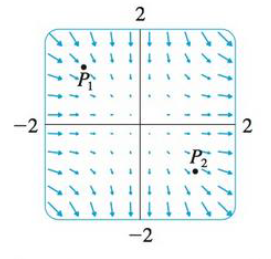Chapter 16.9, Problem 19E

Chapter
Section
Textbook Problem

A vector field F is shown. Use the interpretation of divergence derived in this section to determine whether div F is positive or negative at P1 and at P2.To determine

Whether the divergence of vector field (divF) is positive or negative at P1 and at P2 .

Explanation

If the net flow of the vectors is inward at a point P in the vector field, then the divergence of the vector field at the point P is negative and the point is called sink.

Refer to Figure in the textbook (i.e., bottom of the question 16.9-19E).

From the Figure in the textbook, the vectors enter towards the point P1 are longer than the vectors leaving from the point P1 .

As the vectors that end near the point P1 are longer than the vectors that start near the point P1 , the net flow of the vectors is inward.

Therefore, the divergence of the vector field is negative at the point P1 .

If the net flow of the vectors is outward at a point P in the vector field, then the divergence of the vector field at the point P is positive and the point is called source

Still sussing out bartleby?

Check out a sample textbook solution.

See a sample solution

The Solution to Your Study Problems

Bartleby provides explanations to thousands of textbook problems written by our experts, many with advanced degrees!

Get Started

Find the mean for the following set of scores: 2, 7, 9, 4, 5, 3, 0, 6

Essentials of Statistics for The Behavioral Sciences (MindTap Course List)

True or False: converges mean exists.

Study Guide for Stewart's Single Variable Calculus: Early Transcendentals, 8th

Given that for all x, a power series for is:

Study Guide for Stewart's Multivariable Calculus, 8th

In Exercises 110, evaluate the expression. 4(18!)

Finite Mathematics for the Managerial, Life, and Social Sciences